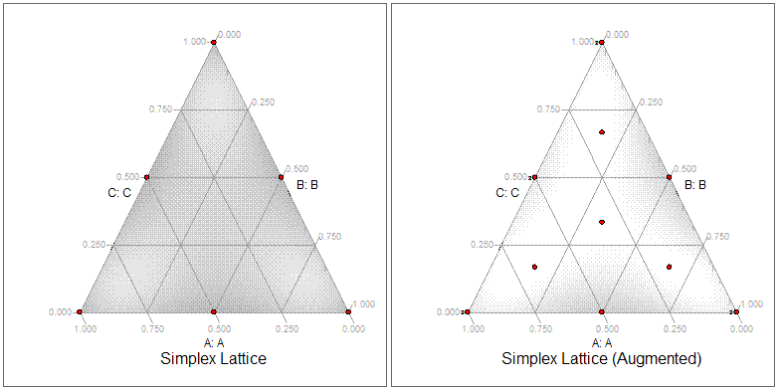# Simplex Lattice Design¶

This design can be used for 2 to 30 components. A simplex-lattice mixture design of degree m consists of m+1 points of equally spaced values between 0 and 1 for each component. If m = 2 then possible fractions are 0, 1/2, 1. For m = 3 the possible values are 0, 1/3, 2/3, 1. The points include the pure components and enough points between them to estimate an equation of degree m. This design differs from a simplex-centroid design by having enough points to estimate a full cubic model.

These designs should be augmented to allow for detection of lack of fit. Note: the software does this for you by default. Simplex-lattice augmentation adds the overall centroid plus all check blends. Check blends are half-way between the center point and each vertex.

To test the lack of fit, an estimate of pure error is necessary. This estimate of pure error comes from replication of several design points. By default, the software adds a number of replications equal to the number of components plus one, up to a maximum of 5.